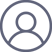文克玲“刻舟求鱼”究竟是怎么回事？
2016-12-20 00:05

“刻舟求鱼”究竟是怎么回事？

1.如果水的阻力严格为零，船+人的系统的质心位置不变，而船会后退一个距离$\delta x$，人前移。

2.如果水的阻力为无穷大，则船完全不动。

3.实际情况在两者之间。

$S(T) = V_0 \frac{m}{M} \frac{M}{k} (e^{-k\ T/M}-1)" original="http://latex.codecogs.com/gif.latex?S(T) = V_0 \frac{m}{M} \frac{M}{k} (e^{-k\ T/M}-1)" style="margin:0px;padding:0px;word-wrap:break-word;max-width:620px;font-family:tahoma, helvetica, simsun, sans-serif, hei;font-size:14px;background-color:#ffffff;display:inline;$

$S^\prime(\infty) = V^\prime \frac{m+M}{k} = V_0 \frac{m}{m+M} (1-e^{-kT/M}) \frac{m+M}{k} \\= V_0 \frac{m}{k} (1-e^{-kT/M})" original="http://latex.codecogs.com/gif.latex?S^\prime(\infty) = V^\prime \frac{m+M}{k} = V_0 \frac{m}{m+M} (1-e^{-kT/M}) \frac{m+M}{k} \\= V_0 \frac{m}{k} (1-e^{-kT/M})" style="margin:0px;padding:0px;word-wrap:break-word;max-width:620px;display:inline;$

$d = S(T) + S^\prime(\infty) =0" original="http://latex.codecogs.com/gif.latex?d = S(T) + S^\prime(\infty) =0" style="margin:0px;padding:0px;word-wrap:break-word;max-width:620px;display:inline;$

$x(t)=-S(T){e^{ - t/\tau }}$                    （1）

$x(t)=-S(T)$                                 （2）